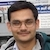# Direction of the Frictional Forces on the Bicycle Wheels during PedalingBy

The friction force acts in the forward direction on the rear wheel and it acts in the backward direction on the front wheel.

The magnitude of friction force on the rear wheel can be more, equal or less than that on the front wheel. The friction force on the rear wheel is more than that on the front wheel if the bicycle accelerates. These two are equal if the bicycle moves with a constant speed. The friction force on the rear wheel is less than that on the front wheel if the bicycle decelerate.

If pedaling is stopped, the direction of friction force on the rear wheel gets reversed.

## Friction Force on the Front Wheel

The front wheel is connected to the rest of the bicycle by a rod passing through its centre (axle). The torque on the wheel about its centre by the force coming from the rest of the bicycle is zero. Thus, pedaling can give linear velocity to the front wheel but cannot rotate it.

Let us analyse a simple situation to get the direction of friction force on the front wheel. What will happen if a wheel translating with speed $u$ is gently placed on a rough surface? Every point on the wheel, including centre C and contact point P translates with the same speed $u$. Thus, P moves towards right relative to the surface. To oppose this, frictional force at P acts towards the left. The frictional force retards the velocity of the centre. The torque about C due to frictional force gives clockwise angular acceleration and the wheel starts rotating in clockwise direction. If the coefficient of friction is sufficiently large then the wheel will start rolling without slipping after some time. At this instant, velocity of P relative to the surface becomes zero. The frictional force will become zero and the wheel continues to roll without slipping, $v=\omega r$.

## Friction Force on the Rear Wheel

The rear wheel is connected to rest of the bicycle by a rod passing through its centre and a chain connected to the pedals (see figure). Pressing the pedal increases tension in the upper portion of the chain. This tension gives rise to clockwise torque and wheel starts rotating in clockwise direction.Motion of a Rear Wheel on a Rough Horizontal Surface during Pedaling

Let us analyse a simple situation. What will happen if a wheel rotating with angular velocity $\omega_0$ is gently placed on a rough surface? Initial velocity of the contact point P is leftward relative to the surface. To oppose this, frictional force at P acts towards the right. The frictional force increases the velocity of C. The torque about C due to frictional force is anti-clockwise. This torque gives anti-clockwise angular acceleration. If the coefficient of friction is sufficiently large then the wheel will start rolling without slipping after some time. At this instant, velocity of P relative to the surface becomes zero. After this, the wheel continues to roll without slipping, $v=\omega r$.

## More interesting questions?

1. In a real bicycle, the normal force on the front wheel from the ground acts slightly away from the vertical line passing through its centre. The average normal force on the front wheel acts
B. behind this line.
2. A free wheel is rolling without slipping on a rough horizontal surface. The magnitude and direction of the frictional force on the wheel are
A. non-zero, backward.
B. non-zero, forward.
C. zero, undefined.
3. What is the direction of friction force if a free wheel rolls without slipping down an inclined plane?
A. forward.
B. backward.
4. Why the cycle stops if pedaling is stopped?